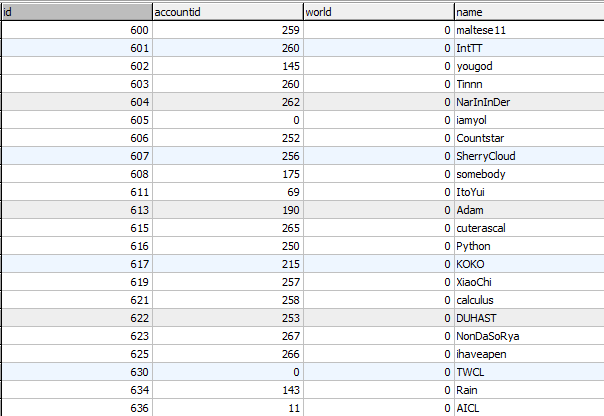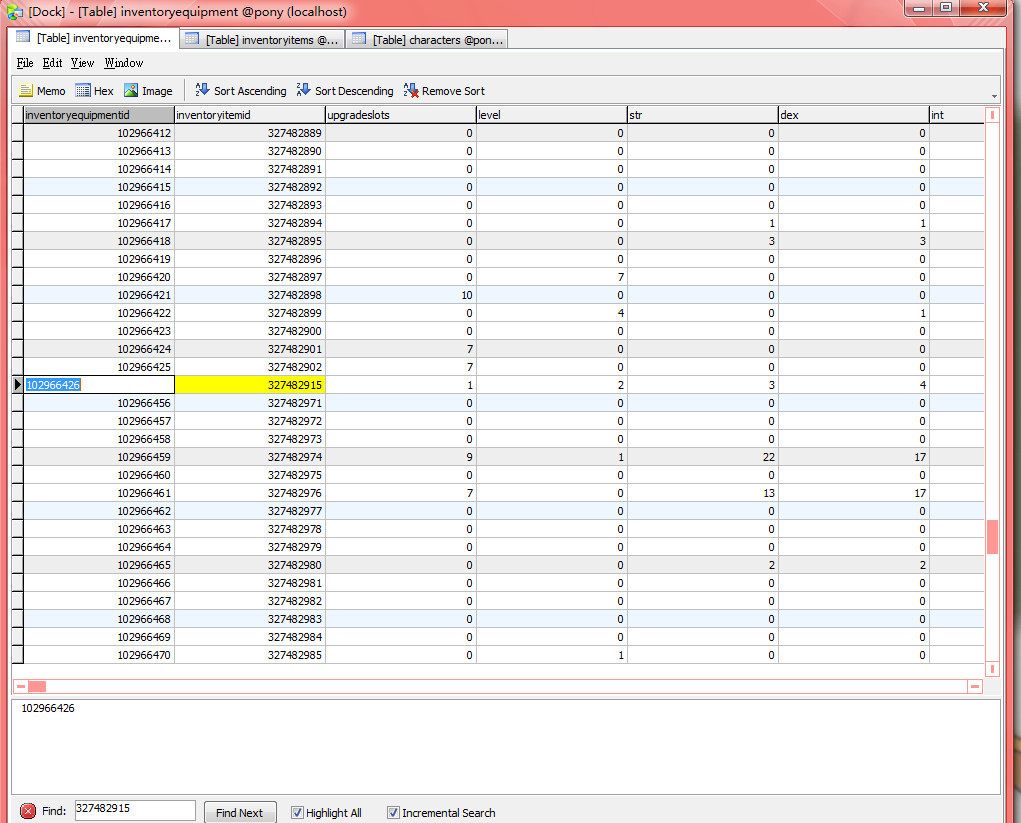## 【楓之谷教學】如何從SQL修改角色裝備各項素質【問題】

【解決】id = 角色編號
accountid = 角色帳號編號
world = 未知
name = 角色名稱inventoryitemid = 道具編號
characterid = 角色編號
storageind = 尚未得知
itemid = 道具代碼
inventorytype = 道具分類位置
1 = 裝備
2 = 消耗
3 = 裝飾
4 = 其它
5 = 特殊
position = 道具在分類中的順序
quantity = 數量inventoryequipmentid = 此資料表的序列編號
inventoryitemid = 裝備編號
level = 成功衝卷次數
str = 力量
dex = 敏捷
int = 智力
luk = 幸運
hp = 血量
mp = 魔力
watk = 物理攻擊力
matk = 魔法攻擊力
wdef = 物理防禦力
mdef = 魔法防禦力
acc = 命中力
avoid = 迴避力
hands = HANDS
speed = 移動速度
jump = 跳躍力
ringif = -1 為穿著於身上
locked = 是否鎖定
vicious = 尚未得知

#### { 3 留言... read them below or Comment }

1.2.為何我沒有找到locked

1.我自己也沒看到locked,不過經過嘗試,是flag,把這項改成0,1代表鎖著